Get inspired by the success stories of our students in IIT JAM MS, ISI  MStat, CMI MSc DS.  Learn More

# ISI MStat 2018 PSA Problem 10 | Dirichlet FunctionThis is a problem from ISI MStat 2018 PSA Problem 10 based on Dirichlet Function.

## Dirichlet Function - ISI MStat Year 2018 PSA Question 10

Let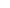be a real number. Then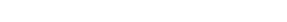• (A) does not exist for any x
• (B) exists for all x
• (C) exists if and only if x is irrational
• (D) exists if and only if x is rational

### Key Concepts

Limit

Sandwich Theorem

ISI MStat 2018 PSA Problem 10

Introduction to Real Analysis by Bertle Sherbert

## Try with Hints

Check two cases separately one when x is rational and other is when x is irrational.

If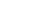is an integer, then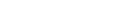If x is rational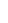, then, eventually, for large enough m, m! will be divisible by q , so thatwill be an integer, and we have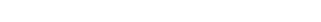If x is irrational,will never be an integer, and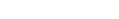, so that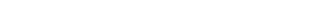for all m>0 by Sandwich Theorem.

## Subscribe to Cheenta at Youtube

This is a problem from ISI MStat 2018 PSA Problem 10 based on Dirichlet Function.

## Dirichlet Function - ISI MStat Year 2018 PSA Question 10

Letbe a real number. Then• (A) does not exist for any x
• (B) exists for all x
• (C) exists if and only if x is irrational
• (D) exists if and only if x is rational

### Key Concepts

Limit

Sandwich Theorem

ISI MStat 2018 PSA Problem 10

Introduction to Real Analysis by Bertle Sherbert

## Try with Hints

Check two cases separately one when x is rational and other is when x is irrational.

Ifis an integer, thenIf x is rational, then, eventually, for large enough m, m! will be divisible by q , so thatwill be an integer, and we haveIf x is irrational,will never be an integer, and, so thatfor all m>0 by Sandwich Theorem.

## Subscribe to Cheenta at Youtube

This site uses Akismet to reduce spam. Learn how your comment data is processed.

### 2 comments on “ISI MStat 2018 PSA Problem 10 | Dirichlet Function”

1.Arnab Chattopadhyay. says:

What I can see that the limit exists for all real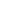Ifis rational the limit evaluates toand ifis irrational the limit evaluates to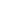Then why do say that the limit doesn't exist for any real? The reasoning is not quite clear to me. Can you please explain?

1.Pravat Hati says:

Don't worry. It will be (B) i.e limit exists for all real x we have also shown that . I mistakenly put A as option . Between thanks for your valuable reply.

### Knowledge Partner